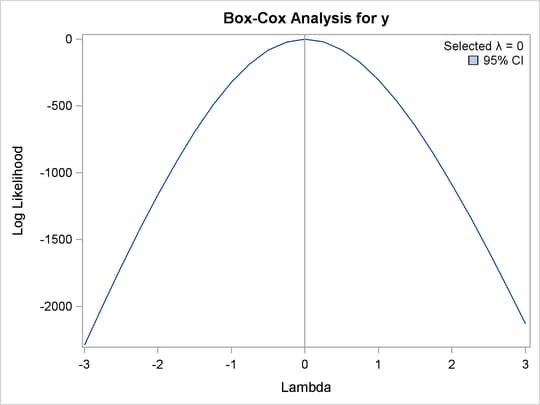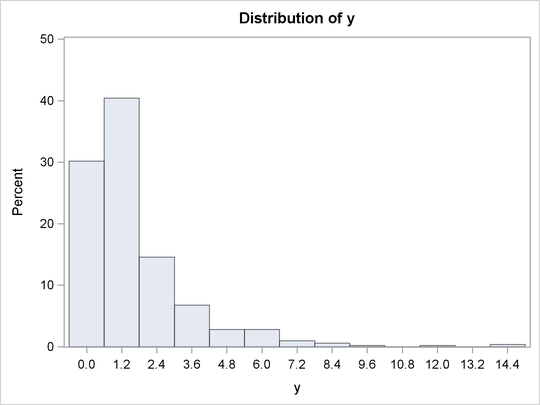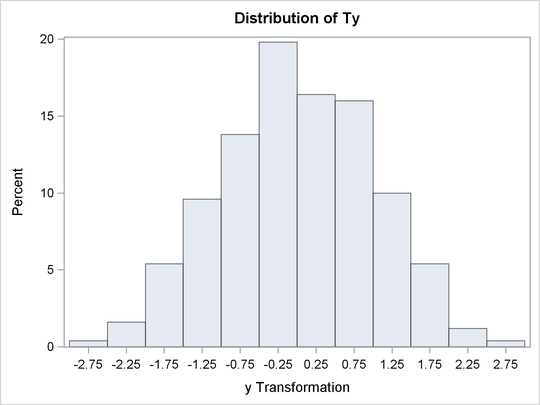Box-Cox Transformations

Box-Cox (1964) transformations are used to find potentially nonlinear transformations of a dependent variable. The Box-Cox transformation has the form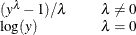This family of transformations of the positive dependent variable y is controlled by the parameter. Transformations linearly related to square root, inverse, quadratic, cubic, and so on are all special cases. The limit asapproaches 0 is the log transformation. More generally, Box-Cox transformations of the following form can be fit: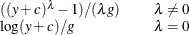By default, c = 0. The parameter c can be used to rescale y so that it is strictly positive. By default, g = 1. Alternatively, g can be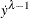, where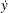is the geometric mean of y.

The BOXCOX transformation in PROC TRANSREG can be used to perform a Box-Cox transformation of the dependent variable. You can specify a list of power parameters by using the LAMBDA= t-option. By default, LAMBDA=–3 TO 3 BY 0.25. The procedure chooses the optimal power parameter by using a maximum likelihood criterion (Draper and Smith 1981, pp. 225–226). You can specify the PARAMETER=c transformation option when you want to shift the values of y, usually to avoid negatives. To divide by, specify the GEOMETRICMEAN t-option.

Here are three examples of using the LAMBDA= t-option:

model BoxCox(y / lambda=0) = identity(x1-x5);
model BoxCox(y / lambda=-2 to 2 by 0.1) = identity(x1-x5);
model BoxCox(y) = identity(x1-x5);

Here is the first example:

model BoxCox(y / lambda=0) = identity(x1-x5);

LAMBDA=0 specifies a Box-Cox transformation with a power parameter of 0. Since a single value of 0 was specified for LAMBDA=, there is no difference between the following models:

model BoxCox(y / lambda=0) = identity(x1-x5);
model log(y) = identity(x1-x5);

Here is the second example:

model BoxCox(y / lambda=-2 to 2 by 0.1) = identity(x1-x5);

LAMBDA= specifies a list of power parameters. PROC TRANSREG tries each power parameter in the list and picks the best transformation. A maximum likelihood approach (Draper and Smith 1981, pp. 225–226) is used. With Box-Cox transformations, PROC TRANSREG finds the transformation before the usual iterations begin. Note that this is quite different from PROC TRANSREG’s usual approach of iteratively finding optimal transformations with ordinary and alternating least squares. It is analogous to SMOOTH and PBSPLINE, which also find transformations before the iterations begin based on a criterion other than least squares.

Here is the third example:

model BoxCox(y) = identity(x1-x5);

The default LAMBDA= list of –3 TO 3 BY 0.25 is used.

The procedure prints the optimal power parameter, a confidence interval on the power parameter (based on the ALPHA= t-option), a convenient power parameter (selected from the CLL= t-option list), and the log likelihood for each power parameter tried (see Example 97.2).

To illustrate how Box-Cox transformations work, data were generated from the model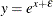where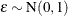. The transformed data can be fit with a linear model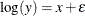The following statements produce Figure 97.14 through Figure 97.15:

title 'Basic Box-Cox Example';

data x;
do x = 1 to 8 by 0.025;
y = exp(x + normal(7));
output;
end;
run;

ods graphics on;

title2 'Default Options';

proc transreg data=x test;
model BoxCox(y) = identity(x);
run;

Figure 97.14: Basic Box-Cox Example, Default Output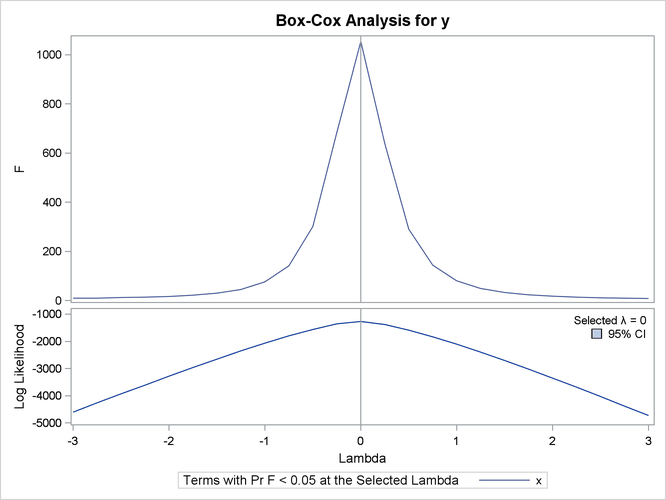Figure 97.14 shows that PROC TRANSREG correctly selects the log transformation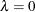, with a narrow confidence interval. The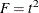plot shows that F is at its largest in the vicinity of the optimal Box-Cox transformation.

The rest of the output, which contains the ANOVA results, is shown in Figure 97.15.

Figure 97.15: Basic Box-Cox Example, Default Output

Dependent Variable BoxCox(y)

 Number of Observations Read 281 281

The TRANSREG Procedure Hypothesis Tests for BoxCox(y)

Univariate ANOVA Table Based on the Usual Degrees of Freedom
Source DF Sum of Squares Mean Square F Value Liberal p
Model 1 1145.884 1145.884 1053.66 >= <.0001
Error 279 303.421 1.088
Corrected Total 280 1449.305

 Root MSE R-Square 1.04285 0.7906 4.49653 0.7899 23.1923 0

This next example uses several options. The LAMBDA= t-option specifies power parameters sparsely from –2 to –0.5 and 0.5 to 2 just to get the general shape of the log-likelihood function in that region. Between –0.5 and 0.5, more power parameters are tried. The CONVENIENT t-option is specified so that if a power parameter like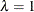oris found in the confidence interval, it is used instead of the optimal power parameter. PARAMETER=2 is specified to add 2 to each y before performing the transformations. ALPHA=0.00001 specifies a wide confidence interval.

These next statements perform the Box-Cox analysis and produce Figure 97.16 and Figure 97.17:

title2 'Several Options Demonstrated';

proc transreg data=x ss2 details
plots=(transformation(dependent) scatter
observedbypredicted);
model BoxCox(y / lambda=-2 -1 -0.5 to 0.5 by 0.05 1 2
convenient parameter=2 alpha=0.00001) =
identity(x);
run;

Figure 97.16: Basic Box-Cox Example, Several Options Demonstrated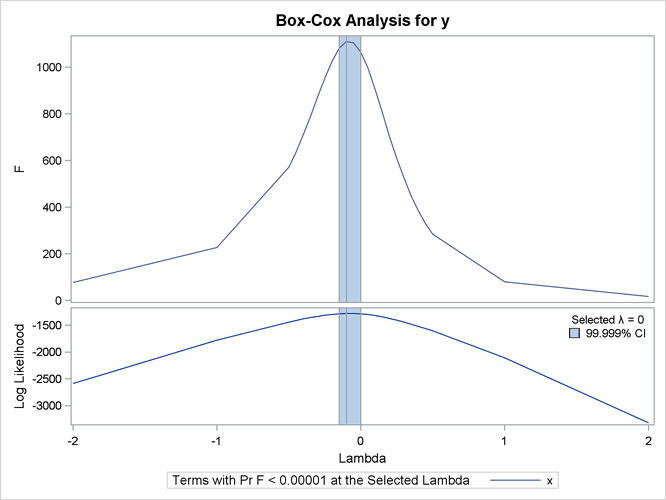The results in Figure 97.16 and Figure 97.17 show that the optimal power parameter is –0.1, but 0 is in the confidence interval, and hence a log transformation is chosen. The actual Box-Cox transformation, the original scatter plot, and observed by predicted values plot are shown in Figure 97.17.

Figure 97.17: Basic Box-Cox Example, Several Options Demonstrated

Dependent Variable BoxCox(y)

 Number of Observations Read 281 281

Model Statement Specification Details
Type DF Variable Description Value
Dep 1 BoxCox(y) Lambda Used 0
Lambda -0.1
Log Likelihood -1280.1
Conv. Lambda 0
Conv. Lambda LL -1287.7
CI Limit -1289.9
Alpha 0.00001
Parameter 2
Options Convenient Lambda Used
Ind 1 Identity(x) DF 1

The TRANSREG Procedure Hypothesis Tests for BoxCox(y)

Univariate ANOVA Table Based on the Usual Degrees of Freedom
Source DF Sum of Squares Mean Square F Value Liberal p
Model 1 999.438 999.4381 1064.82 >= <.0001
Error 279 261.868 0.9386
Corrected Total 280 1261.306

 Root MSE R-Square 0.96881 0.7924 4.61429 0.7916 20.9959 0

Univariate Regression Table Based on the Usual Degrees of Freedom
Variable DF Coefficient Type II
Sum of
Squares
Mean Square F Value Liberal p
Intercept 1 0.42939328 8.746 8.746 9.32 >= 0.0025
Identity(x) 1 0.92997620 999.438 999.438 1064.82 >= <.0001

The above statistics are not adjusted for the fact that the dependent variable was transformed and so are generally liberal.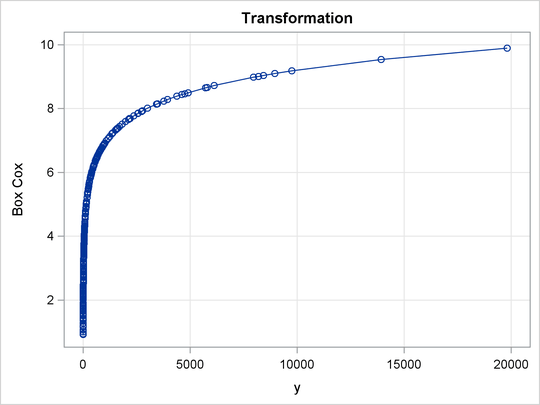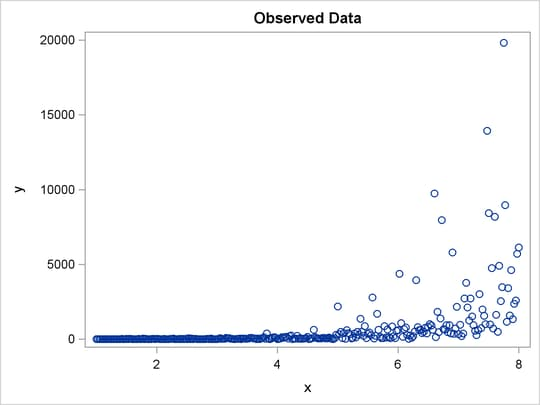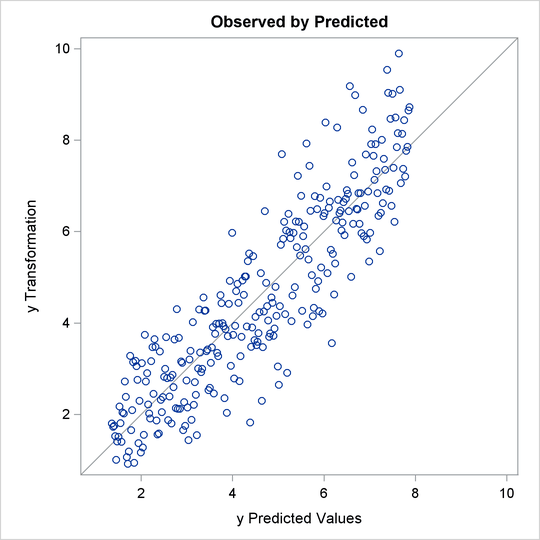The next example shows how to find a Box-Cox transformation without an independent variable. This seeks to normalize the univariate histogram. This example generates 500 random observations from a lognormal distribution. In addition, a constant variable z is created that is all zero. This is because PROC TRANSREG requires some independent variable to be specified, even if it is constant. Two options are specified in the PROC TRANSREG statement. MAXITER=0 is specified because the Box-Cox transformation is performed before any iterations are begun. No iterations are needed since no other work is required. The NOZEROCONSTANT a-option (which can be abbreviated NOZ) is specified so that PROC TRANSREG does not print any warnings when it encounters the constant independent variable. The MODEL statement asks for a Box-Cox transformation of y and an IDENTITY transformation (which does nothing) of the constant variable z. Finally, PROC UNIVARIATE is run to show a histogram of the original variable y, and the Box-Cox transformation, Ty. The following statements fit the univariate Box-Cox model and produce Figure 97.18:

title 'Univariate Box-Cox';

data x;
call streaminit(17);
z = 0;
do i = 1 to 500;
y = rand('lognormal');
output;
end;
run;

proc transreg maxiter=0 nozeroconstant;
model BoxCox(y) = identity(z);
output;
run;

proc univariate noprint;
histogram y ty;
run;

The PROC TRANSREG results in Figure 97.18 show that zero is chosen for lambda, so a log transformation is chosen. The first histogram shows that the original data are skewed, but a log transformation makes the data appear much more nearly normal.

Figure 97.18: Box-Cox with No Independent Variable